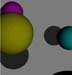# Building a Ray Tracer - Page One

#### © 1996 Harriet Fell for CSG140 (formerly COM3370) Computer Graphics

For simplicity, all scenes are described in screen coordinates. The view plane coincides with the screen plane and the view window goes from (0, 0, 0) in the upper left to (433, 453, 0) in the lower right. The objects (all spheres in these first pictures) are placed between the screen and the viewer. The direction from the screen toward the viewer is the positive z-axis.

[Ambient Light | Diffuse Light | Shadows on Plane | Shadows | Spheres in Space | Next Page ]

##### Only Ambient Light
For each pixel to be colored, a ray is followed to the viewer. The color of the sphere, intersecting that ray, that is nearest to the viewer determines the pixel color. If no sphere is intersected, a medium gray is used. The light source does not affect the scene. There is no shading on the spheres.

View Point = (200, 200, 1000)
Light Source = (750, 0, 2000)
Spheres:
Center = (100, 100, 100) Radius = 50 RGB = (0.5, 0.0, 0.5)
Center = (150, 200, 300) Radius = 50 RGB = (0.5, 0.5, 0.0)
Center = (350, 220, 150) Radius = 50 RGB = (0.0, 0.5, 0.5)
Center = (250, 300, 400) Radius = 50 RGB = (0.25, 0.25, 0.5)

##### Diffuse Light
For each pixel to be colored, a ray is followed to the viewer and the point of intersection with an object that is nearest to the viewer is found. A ray is then followed from that point to the light source and Lambert's Law is applied to determine the shading at that point. The spheres now look 3-dimensional but there are no shadows.

Same data as above.

[Ambient Light | Diffuse Light | Shadows on Plane | Shadows | Spheres in Space | Next Page ]

##### Diffuse Light, Shadows on the Back Plane
For each pixel to be colored, a ray is followed to the viewer. If the ray intersects a sphere, proceed as above. If there is no intersection on the way to the viewer, follow a ray from the pixel to the light source. If that ray intersects a sphere, the pixel is in shadow and should be colored appropriately.

Same data as above.

#### Other views##### Closeup of the Four Spheres

Same spheres as above.

View Point = (200, 200, 500)
Light Source = (500, 250, 1000)

##### Spheres Lying on the Back Plane

View Point = (200, 200, 2000)
Light Source = (500, 250, 1000)
Spheres:
Center = (100, 100, 50) Radius = 50 RGB = (0.5, 0.0, 0.5)
Center = (150, 200, 50) Radius = 50 RGB = (0.5, 0.5, 0.0)
Center = (350, 220, 50) Radius = 50 RGB = (0.0, 0.5, 0.5)
Center = (250, 300, 50) Radius = 50 RGB = (0.25, 0.25, 0.5)

[Ambient Light | Diffuse Light | Shadows on Plane | Shadows | Spheres in Space | Next Page ]

##### Diffuse light, Shadows on the Back Plane and Spheres
For each pixel to be colored, a ray is followed to the viewer. If there is no intersection on the way to the viewer, proceed as above. If the ray to the viewer intersects a sphere, follow a new ray from the nearest point of intersection to the light source. If this ray intersects another sphere, the point is in shadow; if not use Lambert's law as above.

View Point = (240, 248, 5000)
Light Source = (700, 400, 2000)
Spheres:
Center = (100, 100, 50) Radius = 50 RGB = (0.5, 0.0, 0.5)
Center = (150, 200, 250) Radius = 50 RGB = (0.5, 0.5, 0.0)
Center = (350, 220, 500) Radius = 50 RGB = (0.0, 0.5, 0.5)
Center = (250, 300, 750) Radius = 50 RGB = (0.25, 0.25, 0.5)

Same scene and view point as above with Light Source = (240, 600, 2000)

##### Spheres in Space
The entire image area was set to black. For the starry image, random white dots were added. In the ray tracing, unobstructed pixels were left unchanged. The back plane is actually transparent. The gray sphere lies mostly behind the plane.

[Ambient Light | Diffuse Light | Shadows on Plane | Shadows | Spheres in Space | Next Page ]

Harriet J. Fell
College of Computer Science, Northeastern University
360 Huntington Avenue #202WVHCN,
Boston, MA 02115
Internet: fell@ccs.neu.edu
Phone: (617) 373-2198 / Fax: (617) 373-5121

Last Updated: December 20, 2005 10:48 a.m.
The URL for this document is: http://www.ccs.neu.edu/home/fell/COM3370/RayTrace1.html